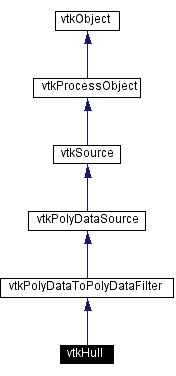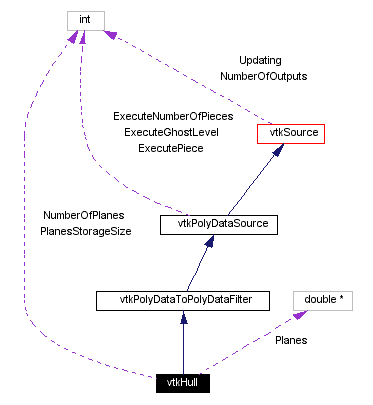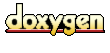Main Page   Class Hierarchy   Alphabetical List   Compound List   File List   Compound Members   File Members   Related Pages

# vtkHull Class Reference

produce an n-sided convex hull. More...

`#include <vtkHull.h>`

Inheritance diagram for vtkHull:[legend]
Collaboration diagram for vtkHull:[legend]
List of all members.

## Public Methods

virtual const char * GetClassName ()
virtual int IsA (const char *type)
void PrintSelf (ostream &os, vtkIndent indent)
void RemoveAllPlanes (void)
int AddPlane (float A, float B, float C)
void SetPlane (int i, float A, float B, float C)
void SetPlane (int i, float plane)
int AddPlane (float A, float B, float C, float D)
int AddPlane (float plane, float D)
void SetPlane (int i, float A, float B, float C, float D)
void SetPlane (int i, float plane, float D)
void SetPlanes (vtkPlanes *planes)
virtual int GetNumberOfPlanes ()
void GenerateHull (vtkPolyData *pd, float *bounds)
void GenerateHull (vtkPolyData *pd, float xmin, float xmax, float ymin, float ymax, float zmin, float zmax)

## Static Public Methods

vtkHull * New ()
int IsTypeOf (const char *type)
vtkHull * SafeDownCast (vtkObject *o)

## Protected Methods

vtkHull ()
~vtkHull ()
vtkHull (const vtkHull &)
void operator= (const vtkHull &)
void ComputePlaneDistances ()
void ClipPolygonsFromPlanes (vtkPoints *points, vtkCellArray *polys, float *bounds)
void CreateInitialPolygon (double *, int, float *)
void Execute ()

## Protected Attributes

double * Planes
int PlanesStorageSize
int NumberOfPlanes

## Detailed Description

produce an n-sided convex hull.

Date:
2001/01/07 14:26:23
Revision:
1.11.2.1

vtkHull is a filter which will produce an n-sided convex hull given a set of n planes. (The convex hull bounds the input polygonal data.) The hull is generated by squeezing the planes towards the input vtkPolyData, until the planes just touch the vtkPolyData. Then, the resulting planes are used to generate a polyhedron (i.e., hull) that is represented by triangles.

The n planes can be defined in a number of ways including 1) manually specifying each plane; 2) choosing the six face planes of the input's bounding box; 3) choosing the eight vertex planes of the input's bounding box; 4) choosing the twelve edge planes of the input's bounding box; and/or 5) using a recursively subdivided octahedron. Note that when specifying planes, the plane normals should point outside of the convex region.

The output of this filter can be used in combination with vtkLODActor to represent a levels-of-detail in the LOD hierarchy. Another use of this class is to manually specify the planes, and then generate the polyhedron from the planes (without squeezing the planes towards the input). The method GenerateHull() is used to do this.

Examples:
vtkHull (examples)

Definition at line 78 of file vtkHull.h.

## Constructor & Destructor Documentation

 vtkHull::vtkHull ( ) ` [protected]`

 vtkHull::~vtkHull ( ) ` [protected]`

 vtkHull::vtkHull ( const vtkHull & ) ` [inline, protected]`
 Definition at line 163 of file vtkHull.h.

## Member Function Documentation

 vtkHull* vtkHull::New ( ) ` [static]`
 Instantiate object with no start, end, or progress methods. Reimplemented from vtkPolyDataSource.

 virtual const char* vtkHull::GetClassName ( ) ` [virtual]`
 Return the class name as a string. This method is defined in all subclasses of vtkObject with the vtkTypeMacro found in vtkSetGet.h. Reimplemented from vtkPolyDataToPolyDataFilter.

 int vtkHull::IsTypeOf ( const char * type ) ` [static]`
 Return 1 if this class type is the same type of (or a subclass of) the named class. Returns 0 otherwise. This method works in combination with vtkTypeMacro found in vtkSetGet.h. Reimplemented from vtkPolyDataToPolyDataFilter.

 virtual int vtkHull::IsA ( const char * type ) ` [virtual]`
 Return 1 if this class is the same type of (or a subclass of) the named class. Returns 0 otherwise. This method works in combination with vtkTypeMacro found in vtkSetGet.h. Reimplemented from vtkPolyDataToPolyDataFilter.

 vtkHull* vtkHull::SafeDownCast ( vtkObject * o ) ` [static]`
 Will cast the supplied object to vtkObject* is this is a safe operation (i.e., a safe downcast); otherwise NULL is returned. This method is defined in all subclasses of vtkObject with the vtkTypeMacro found in vtkSetGet.h. Reimplemented from vtkPolyDataToPolyDataFilter.

 void vtkHull::PrintSelf ( ostream & os, vtkIndent indent ) ` [virtual]`
 Methods invoked by print to print information about the object including superclasses. Typically not called by the user (use Print() instead) but used in the hierarchical print process to combine the output of several classes. Reimplemented from vtkSource.

 void vtkHull::RemoveAllPlanes ( void )
 Remove all planes from the current set of planes.

 int vtkHull::AddPlane ( float A, float B, float C )
 Add a plane to the current set of planes. It will be added at the end of the list, and an index that can later be used to set this plane's normal will be returned. The values A, B, C are from the plane equation Ax + By + Cz + D = 0. This vector does not have to have unit length (but it must have a non-zero length!). If a value 0 > i >= -NumberOfPlanes is returned, then the plane is parallel with a previously inserted plane, and |-i-1| is the index of the plane that was previously inserted. If a value i < -NumberOfPlanes is returned, then the plane normal is zero length.

 int vtkHull::AddPlane ( float plane )

 void vtkHull::SetPlane ( int i, float A, float B, float C )
 Set the normal values for plane i. This is a plane that was already added to the current set of planes with AddPlane(), and is now being modified. The values A, B, C are from the plane equation Ax + By + Cz + D = 0. This vector does not have to have unit length. Note that D is set to zero, except in the case of the method taking a vtkPlanes* argument, where it is set to the D value defined there.

 void vtkHull::SetPlane ( int i, float plane )

 int vtkHull::AddPlane ( float A, float B, float C, float D )
 Variations of AddPlane()/SetPlane() that allow D to be set. These methods are used when GenerateHull() is used.

 int vtkHull::AddPlane ( float plane, float D )

 void vtkHull::SetPlane ( int i, float A, float B, float C, float D )

 void vtkHull::SetPlane ( int i, float plane, float D )

 void vtkHull::SetPlanes ( vtkPlanes * planes )
 Set all the planes at once using a vtkPlanes implicit function. This also sets the D value, so it can be used with GenerateHull().

 virtual int vtkHull::GetNumberOfPlanes ( ) ` [virtual]`
 Get the number of planes in the current set of planes.

 Add the 8 planes that represent the vertices of a cube - the combination of the three face planes connecting to a vertex - (1,1,1), (1,1,-1), (1,-1,1), (1,-1,1), (-1,1,1), (-1,1,-1), (-1,-1,1), (-1,-1-1).

 Add the 12 planes that represent the edges of a cube - halfway between the two connecting face planes - (1,1,0), (-1,-1,0), (-1,1,0), (1,-1,0), (0,1,1), (0,-1,-1), (0,1,-1), (0,-1,1), (1,0,1), (-1,0,-1), (1,0,-1), (-1,0,1)

 Add the six planes that make up the faces of a cube - (1,0,0), (-1, 0, 0), (0,1,0), (0,-1,0), (0,0,1), (0,0,-1)

 void vtkHull::AddRecursiveSpherePlanes ( int level )
 Add the planes that represent the normals of the vertices of a polygonal sphere formed by recursively subdividing the triangles in an octahedron. Each triangle is subdivided by connecting the midpoints of the edges thus forming 4 smaller triangles. The level indicates how many subdivisions to do with a level of 0 used to add the 6 planes from the original octahedron, level 1 will add 18 planes, and so on.

 void vtkHull::GenerateHull ( vtkPolyData * pd, float * bounds )
 A special method that is used to generate a polyhedron directly from a set of n planes. The planes that are supplied by the user are not squeezed towards the input data (in fact the user need not specify an input). To use this method, you must provide an instance of vtkPolyData into which the points and cells defining the polyhedron are placed. You must also provide a bounding box where you expect the resulting polyhedron to lie. This can be a very generous fit, it's only used to create the initial polygons that are eventually clipped.

 void vtkHull::GenerateHull ( vtkPolyData * pd, float xmin, float xmax, float ymin, float ymax, float zmin, float zmax )

 void vtkHull::operator= ( const vtkHull & ) ` [inline, protected]`
 Definition at line 164 of file vtkHull.h.

 void vtkHull::ComputePlaneDistances ( ) ` [protected]`

 void vtkHull::ClipPolygonsFromPlanes ( vtkPoints * points, vtkCellArray * polys, float * bounds ) ` [protected]`

 void vtkHull::CreateInitialPolygon ( double * , int , float * ) ` [protected]`

 void vtkHull::Execute ( ) ` [protected, virtual]`
 Reimplemented from vtkSource.

## Member Data Documentation

 double* vtkHull::Planes` [protected]`
 Definition at line 167 of file vtkHull.h.

 int vtkHull::PlanesStorageSize` [protected]`
 Definition at line 172 of file vtkHull.h.

 int vtkHull::NumberOfPlanes` [protected]`
 Definition at line 175 of file vtkHull.h.

The documentation for this class was generated from the following file:

Generated on Wed Nov 21 12:48:12 2001 for VTK by1.2.11.1 written by Dimitri van Heesch, © 1997-2001